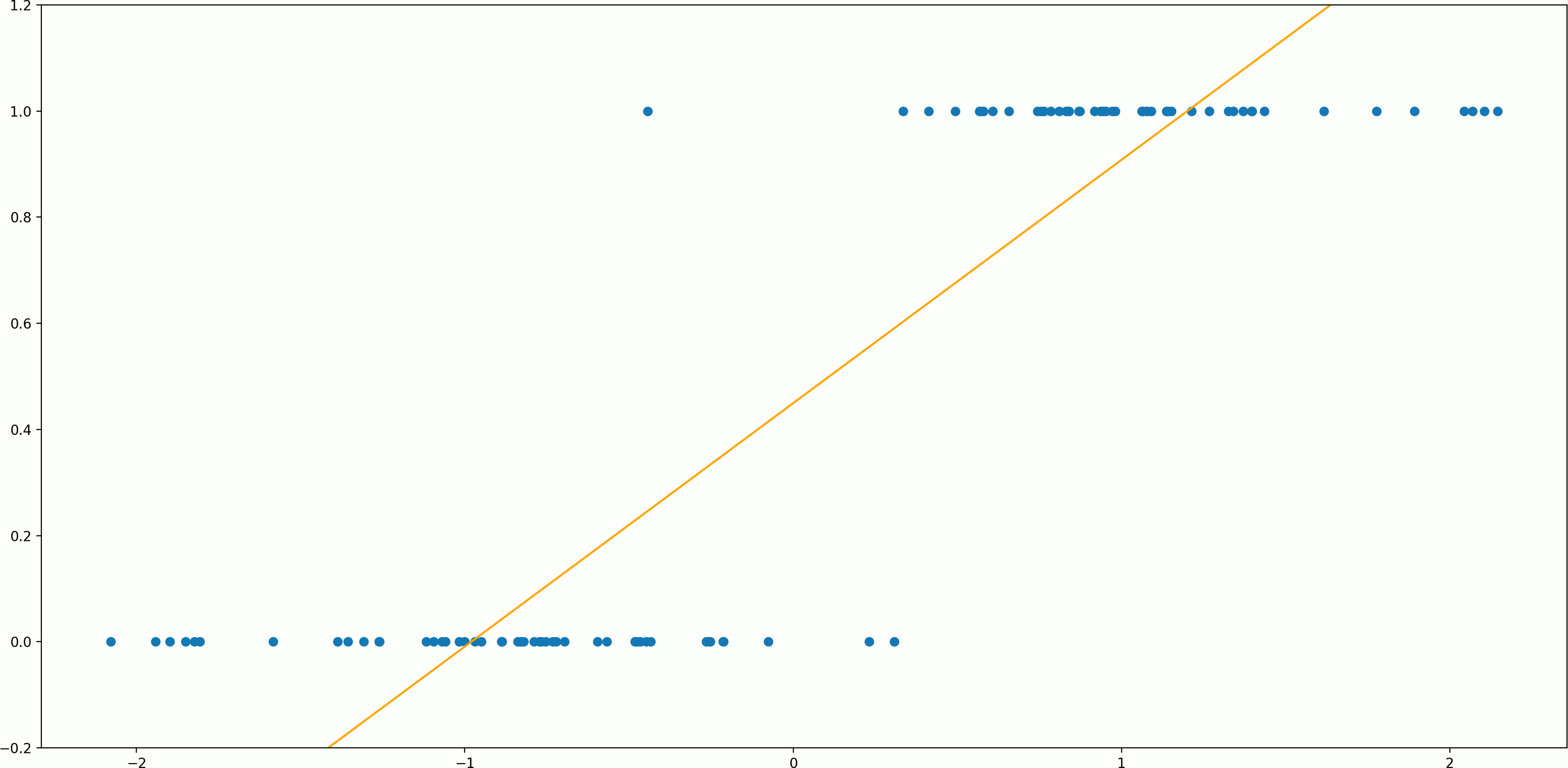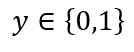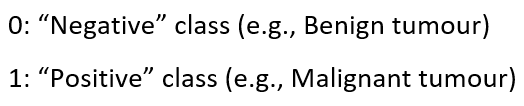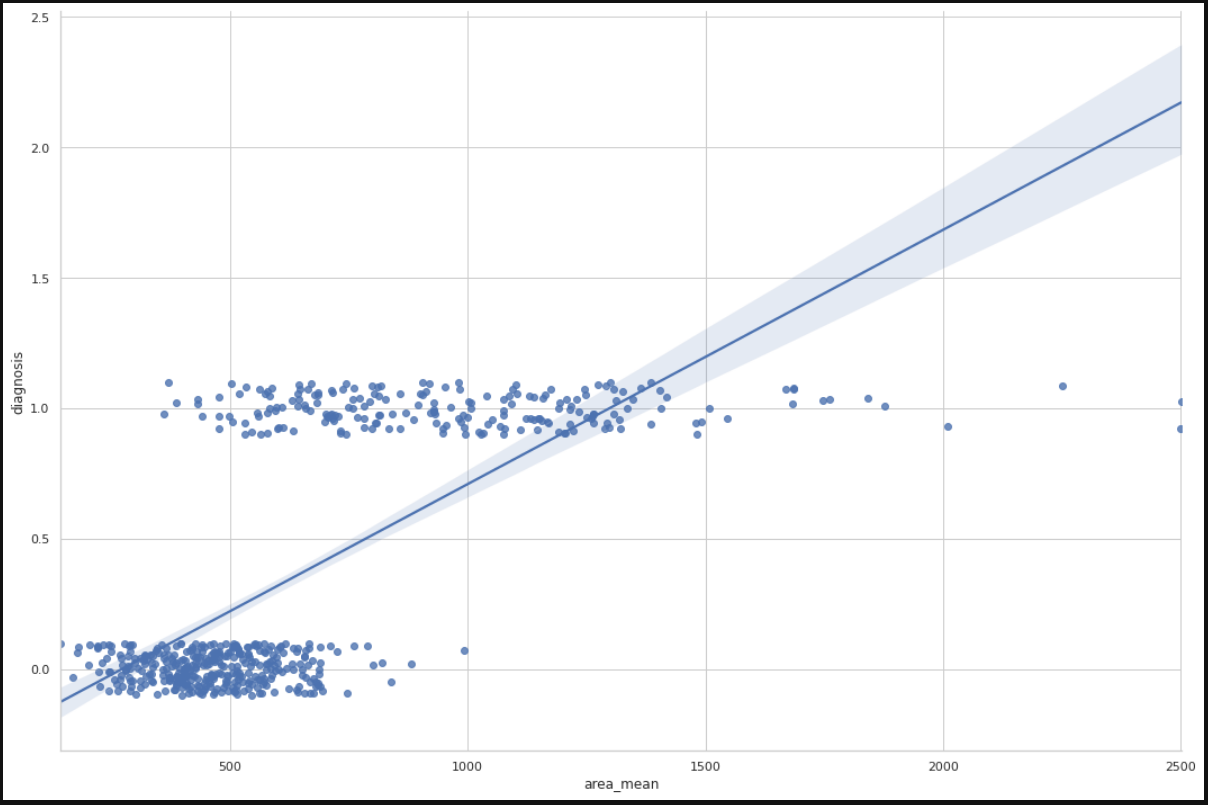1603342800

Understanding Logistic Regression

What is logistic regression? Logistic regression is just adapting linear regression to a special case where you can have only 2 outputs: 0 or 1. And this thing is most commonly applied to classification problems where 0 and 1 represent two different classes and we want to distinguish between them.

Linear regression outputs a real number that ranges from -∞ to +∞. And we can use just this even in the 0/1 classification problem: if we get a value >= 0.5 report it as class label 1, if the output is < 0.5 report it as a 0.Where x is a vector of features (plus a component with constant 1 for bias) of one observation, and w is the weights vector.

But, we can get slightly better results both in terms of accuracy and interpretability if we squash the regression line into an “S”-shaped curve between 0 and 1. We squash the regression line by applying the sigmoid function to the output value of a linear regression model.

Below is the sigmoid function:More exactly, we compute the output as follows: take the weighted sum of the inputs, and then pass this resulting number into the sigmoid function and report the sigmoid’s output as the output of our logistic regression model.

This procedure helps us both in getting slightly better accuracy and in the interpretability of the output. If our model outputs any real number like -5 or 7, what these numbers actually mean? What can we tell about our two classes: 0 and 1?

But, when we have outputs between 0 and 1, we can interpret them as probabilities. The output of a logistic regression model is the probability of our input belonging to the class labeled with 1. And the complement of our model’s output is the probability of our input belonging to the class labeled with 0.

#machine-learning #data-science #artificial-intelligence #mathematics #logistic-regression

Buddha Community1598352300

Regression: Linear Regression

Machine learning algorithms are not your regular algorithms that we may be used to because they are often described by a combination of some complex statistics and mathematics. Since it is very important to understand the background of any algorithm you want to implement, this could pose a challenge to people with a non-mathematical background as the maths can sap your motivation by slowing you down.In this article, we would be discussing linear and logistic regression and some regression techniques assuming we all have heard or even learnt about the Linear model in Mathematics class at high school. Hopefully, at the end of the article, the concept would be clearer.

**Regression Analysis **is a statistical process for estimating the relationships between the dependent variables (say Y) and one or more independent variables or predictors (X). It explains the changes in the dependent variables with respect to changes in select predictors. Some major uses for regression analysis are in determining the strength of predictors, forecasting an effect, and trend forecasting. It finds the significant relationship between variables and the impact of predictors on dependent variables. In regression, we fit a curve/line (regression/best fit line) to the data points, such that the differences between the distances of data points from the curve/line are minimized.

#regression #machine-learning #beginner #logistic-regression #linear-regression #deep learning1600123860

Linear Regression VS Logistic Regression (MACHINE LEARNING)

Linear Regression and Logistic Regression are** two algorithms of machine learning **and these are mostly used in the data science field.

Linear Regression:> It is one of the algorithms of machine learning which is used as a technique to solve various use cases in the data science field. It is generally used in the case of continuous output. For e.g if ‘Area’ and ‘Bhk’ of the house is given as an input and we have found the ‘Price’ of the house, so this is called a regression problem.

Mechanism:> In the diagram below X is input and Y is output value.

#machine-learning #logistic-regression #artificial-intelligence #linear-regression1592023980

5 Regression algorithms: Explanation & Implementation in Python

Take your current understanding and skills on machine learning algorithms to the next level with this article. What is regression analysis in simple words? How is it applied in practice for real-world problems? And what is the possible snippet of codes in Python you can use for implementation regression algorithms for various objectives? Let’s forget about boring learning stuff and talk about science and the way it works.

#linear-regression-python #linear-regression #multivariate-regression #regression #python-programming1597019820

Introduction:

In this article, I will be explaining how to use the concept of regression, in specific logistic regression to the problems involving classification. Classification problems are everywhere around us, the classic ones would include mail classification, weather classification, etc. All these data, if needed can be used to train a Logistic regression model to predict the class of any future example.

Context:

1. Introduction to classification problems.
2. Logistic regression and all its properties such as hypothesis, decision boundary, cost, cost function, gradient descent, and its necessary analysis.
3. Developing a logistic regression model from scratch using python, pandas, matplotlib, and seaborn and training it on the Breast cancer dataset.
4. Training an in-built Logistic regression model from sklearn using the Breast cancer dataset to verify the previous model.

Introduction to classification problems:

Classification problems can be explained based on the Breast Cancer dataset where there are two types of tumors (Benign and Malignant). It can be represented as:whereThis is a classification problem with 2 classes, 0 & 1. Generally, the classification problems have multiple classes say, 0,1,2 and 3.

Dataset:

Breast Cancer Wisconsin (Diagnostic) Data Set

Predict whether the cancer is benign or malignant

www.kaggle.com

1. Let’s import the dataset to a pandas dataframe:
import pandas as pd

2. The following dataframe is obtained:

![Image for post](https://miro.medium.com/max/1750/1*PPyiGgocvjHbgIcs9yTWTA.png)

df.info()

Data analysis:

Let us plot the mean area of the clump and its classification and see if we can find a relation between them.

import matplotlib.pyplot as plt
import seaborn as sns
from sklearn import preprocessing
label_encoder = preprocessing.LabelEncoder()
df.diagnosis = label_encoder.fit_transform(df.diagnosis)
sns.set(style = 'whitegrid')
sns.lmplot(x = 'area_mean', y = 'diagnosis', data = df, height = 10, aspect = 1.5, y_jitter = 0.1)We can infer from the plot that most of the tumors having an area less than 500 are benign(represented by zero) and those having area more than 1000 are malignant(represented by 1). The tumors having a mean area between 500 to 1000 are both benign and malignant, therefore show that the classification depends on more factors other than mean area. A linear regression line is also plotted for further analysis.

#machine-learning #logistic-regression #regression #data-sceince #classification #deep learning1622617140

Logistic Regression -Beginners Guide in Python - Analytics India Magazine

Most of the supervised learning problems in machine learning are classification problems. Classification is the task of assigning a data point with a suitable class. Suppose a pet classification problem. If we input certain features, the machine learning model will tell us whether the given features belong to a cat or a dog. Cat and dog are the two classes here. One may be numerically represented by 0 and the other by 1. This is specifically called a binary classification problem. If there are more than two classes, the problem is termed a multi-class classification problem. This machine learning task comes under supervised learning because both the features and corresponding class are provided as input to the model during training. During testing or production, the model predicts the class given the features of a data point.

This article discusses Logistic Regression and the math behind it with a practical example and Python codes. Logistic regression is one of the fundamental algorithms meant for classification. Logistic regression is meant exclusively for binary classification problems. Nevertheless, multi-class classification can also be performed with this algorithm with some modifications.

#developers corner #binary classification #classification #logistic regression #logit #python #regression #scikit learn #sklearn #statsmodels #tutorial